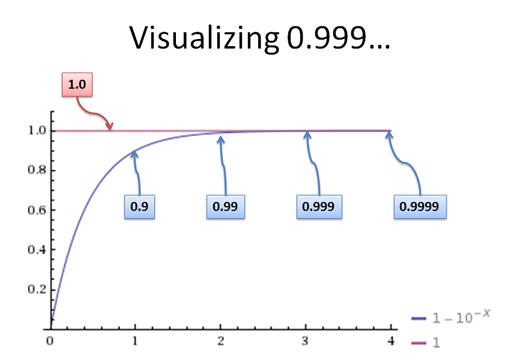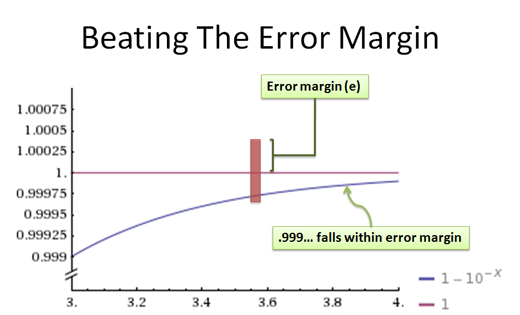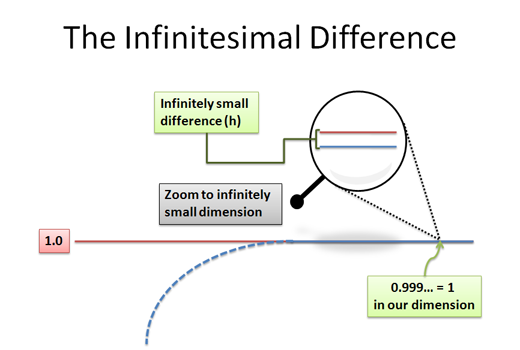# A Friendly Chat About Whether 0.999… = 1

Does .999… = 1? The question invites the curiosity of students and the ire of pedants. A famous joke illustrates my point:

A man is lost at sea in a hot air balloon. He sees a lighthouse approaching in the fog. “Where am I?” he shouts desperately through the wind. “You’re in a balloon!” he hears as he drifts off into the distance.

The response is correct but unhelpful. When people ask about 0.999… they aren’t saying “Hey, could you find the limit of a convergent series under the axioms of the real number system?” (Really? Yes, Really!)

No, there’s a broader, more interesting subtext: What happens when one number gets infinitely close to another?

It’s a rare thing when people wonder about math: let’s use the opportunity! Instead of bluntly offering technical definitions to satisfy some need for rigor, let’s allow ourselves to explore the question.

Here’s my quick summary:

• The meaning of 0.999… depends on our assumptions about how numbers behave.
• A common assumption is that numbers cannot be “infinitely close” together — they’re either the same, or they’re not. With these rules, 0.999… = 1 since we don’t have a way to represent the difference.
• If we allow the idea of “infinitely close numbers”, then yes, 0.999… can be less than 1.

Math can be about questioning assumptions, pushing boundaries, and wondering “What if?”. Let’s dive in.

## Do Infinitely Small Numbers Exist?

The meaning of 0.999… is a tricky concept, and depends on what we allow a number to be. Here’s an example: Does “3 – 4” mean anything to you?

Sure, it’s -1. Duh. But the question is only simple because you’ve embraced the advanced idea of negatives: you’re ok with numbers being less than nothing. In the 1700s, when negatives were brand new, the concept of “3-4” was eyed with great suspicion, if allowed at all. (Geniuses of the time thought negatives “wrapped around” after you passed infinity.)

Infinitely small numbers face a similar predicament today: they’re new, challenge some long-held assumptions, and are considered “non-standard”.

## So, Do Infinitesimals Exist?

Well, do negative numbers exist? Negatives exist if you allow them and have consistent rules for their use.

Our current number system assumes the long-standing Archimedean property: if a number is smaller than every other number, it must be zero. More simply, infinitely small numbers don’t exist.

The idea should make sense: numbers should be zero or not-zero, right? Well, it’s “true” in the same way numbers must be there (positive) or not there (zero) — it’s true because we’ve implicitly excluded other possibilities.

But, it’s no matter — let’s see where the Archimedean property takes us.

## The Traditional Approach: 0.999… = 1

If we assume infinitely small numbers don’t exist, we can show 0.999… = 1.

First off, we need to figure out what 0.999… means. Most mathematicians see the problem like this:

• 0.999… represents a series of numbers: 0.9, 0.99, 0.999, 0.9999, and so on
• The question: does this series get so close (converge) to a result that we cannot tell it apart?

This is the reasoning behind limits: Does our “thing to examine” get so darn close to another number that we can’t tell them apart, no matter how hard we try?

“Well,” you say, “How do you tell numbers apart?”. Great question. The simplest way to compare is to subtract:

• if a – b = 0, they’re the same
• if a – b is not zero, they’re different

The idea behind limits is to find some point at which “a – b” becomes zero (less than any number); that is, we can’t tell the “number to test” and our “result” as different.

## The Error Tolerance

It’s still tough to compare items when they take such different forms (like an infinite series). The next clever idea behind limits: define an error tolerance:

• You give me your tolerance for error / accuracy level (call it “e”)
• I’ll see whether I can get the two things to fall within that tolerance
• If so, they’re equal! If we can’t tell them apart, no matter how hard we try, they must be the same.

Suppose I sell you a raisin granola bar, claiming it’s 100 grams. You take it home, examine the non FDA-approved wrapper, and decide to see if I’m lying. You put the snack on your scale and it shows 100 grams. The scale is accurate to 1 gram. Did I trick you?

You couldn’t know: as far as you can tell, within your accuracy, the granola bar is indeed 100 grams. Our current problem is similar: I’m selling you a “granola bar” weighing 1 gram, but sneaky me, I’m actually giving you one weighing 0.999… grams. Can you tell the difference?

Ok, let’s work this out. Suppose your error tolerance is 0.1 gram. Then if you ask for 1, and I give you 0.99, the difference is 0.01 (one hundredth) and you don’t know you’ve been tricked! 1 and .99 look the same to you.

But that’s child’s-play. Let’s say your scale is accurate to 1e-9 (.000000001, a billionth of a gram). Well then, I’ll sell you a candy bar that is .999999999999 (only one trillionth of a gram off) and you’ll be fooled again! Hah!

In fact, instead of picking a specific tolerance like 0.01, let’s use a general one (e):

• Error tolerance: e
• Difference: Well, suppose e has “n” digits of precision. Let 0.999… expand until we have a difference requiring n+1 digits of precision to detect.
• Therefore, the tolerance can always be less than e! And the difference appears to be zero.

See the trick? Here’s a visual way to represent it:The straight line is what you’re expecting: 1.0, that perfect granola bar. The curve is the number of digits we expand 0.999… to. The idea is to expand 0.999… until it falls within “e”, your tolerance:At some point, no matter what you pick for e, 0.999… will get close enough to satisfy us mathematically.

(As an aside, 0.999… isn’t a growing process, it’s a final result on its own. The curve represents the idea that we can approximate 0.999… with better and better accuracy — this is fodder for another post).

With limits, if the difference between two things is smaller than any margin we can dream of, they must be the same.

## Assuming Infinitesimals Exist

This first conclusion may not sit well with you — you might feel tricked. And that’s ok! We seem to be ignoring something important when we say that 0.999… equals 1 because we, with our finite precision, cannot tell the difference.

Newer number systems have developed the idea that infinitesimals exist. Specifically:

• Infinitely small numbers can exist: they aren’t zero, but look like zero to us.

This seems to be a confusing idea, but I see it like this: atoms don’t exist to cavemen. Once they’ve cut a rock into grains of sand, they can go no further: that’s the smallest unit they can imagine. Things are either grains, or not there. They can’t imagine the concept of atoms too small for the naked eye.

Compared to other number systems, we’re cavemen. What we call “tiny numbers” are actually gigantic. In fact, there can be another “dimension” of numbers too small for us to detect — numbers that differ only in this tiny dimension look identical to us, but are different under an infinitely powerful microscope.

I interpret 0.999… like this: Can we make a number a bit less than 1 in this new, infinitely small dimension?

## Hyperreal Numbers

Hyperreal numbers are one system that uses this “tiny dimension” to examine infinitely small numbers. In this, infinitesimals are usually called “h”, and are considered to be 1/H (where big H is infinity).

So, the idea is this:

• 0.999… < 1 [We’re assuming it’s allowed to be smaller, and infinitely small numbers exist]
• 0.999… + h = 1 [h is the infinitely small number that makes up the gap]
• 0.999… = 1 – h [Equivalently, we can subtract an infinitely small amount from 1]

So, 0.999… is just a tiny bit less than 1, and the difference is h!

## Back to Our Numbers

The problem is, “h” doesn’t exist back in our macroscopic world. Or rather, h looks the same as zero to us — we can’t tell that it’s a tiny atom, not the lack of any matter altogether. Here’s one way to visualize it:When we switch back to our world, it’s called taking the “standard part” of a number. It essentially means we throw away all the h’s, and convert them to zeroes. So,

• 0.999… = 1 – h [there is an infinitely small difference]
• St(0.999…) = St(1 – h) = St(1) – St(h) = 1 – 0 = 1 [And to us, 0.999… = 1]

The happy compromise is this: in a more accurate dimension, 0.999… and 1 are different. But, when we, with our finite accuracy, try to describe the difference, we cannot: 0.999… and 1 look identical.

## Lessons Learned

Let’s hop back to our world. The purpose of “Does 0.999… equal 1?” is not to spit back the answer to a limit question. That’s interpreting the query as “Hey, within our system what does 0.999… represent?”

The question is about exploration. It’s really, “Hey, I’m wondering about numbers infinitely close together (.999… and 1). How do we handle them?”

Here’s my response:

• Our idea of a number has evolved over thousands of years to include new concepts (integers, decimals, rationals, reals, negatives, imaginary numbers…).
• In our current system, we haven’t allowed infinitely small numbers. As a result, 0.999… = 1 because we don’t allow there to be a gap between them (so they must be the same).
• In other number systems (like the hyperreal numbers), 0.999… is less than 1. Here, infinitely small numbers are allowed to exist, and this tiny difference (h) is what separates 0.999… from 1.

There are life lessons here: can we extend our mental model of the world? Negatives gave us the conception that every number can have an opposite. And you know what? It turns out matter can have an opposite too (matter and antimatter annihilate each other when they come in contact, just like 3 + (-3) = 0).

Let’s think about infinitesimals, a tiny dimension beyond our accuracy:

• Some theories of physics reference tiny “curled up” dimensions which are embedded into our own. These dimensions may be infinitely small compared to our own — we never notice them. To me, “infinitely small dimensions” are a way to describe something which is there, but undetectable to us.
• The physical sciences use “significant figures” and error margins to specify the inherent inaccuracy of our calculations. We know that reality is different from what we actually measure: infinitesimals help make this distinction explicit.
• Making models: An infinitely small dimension can help us create simple but accurate models to solve problems in our world. The idea of “simple but accurate enough” is at the heart of calculus.

Math isn’t just about solving equations. Expanding our perspective with strange new ideas helps disparate subjects click. Don’t be afraid wonder “What if?”.

## Appendix: Where’s the Rigor?

When writing, I like to envision a super-pedant, concerned more with satisfying (and demonstrating) his rigor than educating the reader. This mythical(?) nemesis inspires me to focus on intuition. I really should give Mr. Rigor a name.

But, rigor has a use: it helps ink the pencil-lines we’ve sketched out. I’m not a mathematician, but others have written about the details of interpreting 0.999… and 1 or less than 1:

“So long as the number system has not been specified, the students’ hunch that .999… can fall infinitesimally short of 1, can be justified in a mathematically rigorous fashion.”

My goal is to educate, entertain, and spread interest in math. Can you think of a more salient way to get non-math majors interested in the ideas behind analysis? Limits aren’t going to market themselves.